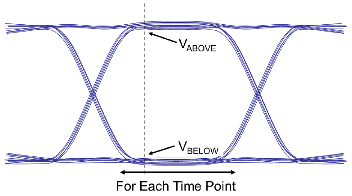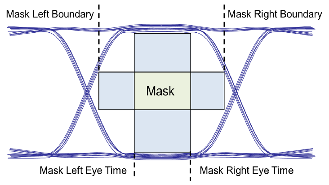## Waveform Reports

The Waveform and Timing window report contains multiple tabs with waveform data and simulation results. The results include syntax errors, analysis warnings and failures, violations of DRC and overshoot rules, waveform quality margins, and eye diagram details.

### Waveform Analysis Report Tabs

#### Waveform Analysis Log

The Waveform Analysis Log tab contains syntax errors in the data and a summary of the waveform analysis failures.

#### Waveform Summary

The Waveform Summary tab summarizes the errors and violations reported by the Waveform Fatal tab, Waveform Quality tab, and Waveform Overshoot tab. The spreadsheet contains one row per transfer net.

#### Waveform Fatal

The Waveform Fatal tab contains all fatal waveform processing errors. A fatal error on an edge prevents the app from generating any timing or waveform data for that edge.

Note

This tab only appears if there is a violation.

#### Waveform Quality

The Waveform Quality tab contains all waveform DRC warnings. A warning indicates that either a waveform DRC rule has been violated or one of the basic waveform transition rules has been broken.

Note

This tab only appears if there is a violation.

#### Waveform Overshoot

The Waveform Overshoot tab contains all violations of waveform overshoot rules.

Note

This tab only appears if there is a violation.

#### Waveform Margin by Variation

The Waveform Margin tab contains all salient waveform quality information that appears under the other waveform quality tabs by simulation. For pre-layout, it includes the solution space values. The margins are reported as one row per simulation.

#### Eye Details

The Eye Details tab contains detailed data on the eye diagram at the receiver for each simulation. This includes eye opening with timing jitter and the outer amplitudes of its envelope. This tab also has the timing points on the simulation where this data was measured. For pre-layout, it includes the solution space values. The eye measurements are reported as one row per node per simulation.

#### Eye Rollups

The Eye Rollups tab contains the eye diagram, eye opening, jitter and outer height by receiver. It summarizes the eye details for each transfer net.

#### Waveform Margin by TNET

The Waveform Margin by TNET tab contains waveform quality information that appears under the other waveform quality tabs, organized by simulation and rolled up for each transfer net. The margins are reported as one row per transfer net.

#### Derating Details

The Derating Details tab contains the slew rates and the etch delays (raw and derated). The slew rate parameters are measured as:

• Slew Rate (rising) — Last crossing of `Vin_DC_Low` to first crossing of `Vin_AC_High`.

• Slew Rate (falling) — Last crossing of `Vin_DC_High` to first crossing of `Vin_AC_Low`.

• DC Slew Rate (rising) — Last crossing of `Vin_DC_Low` to first crossing of `Vin_DC_High`.

• DC Slew Rate (falling) — Last crossing of `Vin_DC_High` to first crossing of `Vin_DC_Low`.

• AC Slew Rate (rising) — Last crossing of `Vmeas` to first crossing of `Vin_AC_High`.

• AC Slew Rate (falling) — Last crossing of `Vmeas` to first crossing of `Vin_AC_Low`.

The Parallel Link Designer app processes the mask data waveforms individually and combine them to create the mask reports. mask does not have fixed measurement thresholds. All measurements are relative to `Vref`. The app determines `Vref` for each waveform and then combines them for each memory device.

# Eyes Rolled up For Margin
• The number of eyes the app uses to determine the margins.

• It can be more than one memory device.

# Eyes Rolled up For Vref
• The number of eyes the app uses to determine the trained VREF.

• Typically all eye for a memory device.

Trained Vref (V)Halfway between the smallest Mask High Boundary and largest Mask Low Boundary of all eye rolled up for VREF.
Trained Vref (%)

Defined as:

`$100\cdot \frac{{\text{V}}_{\text{REF}}}{{\text{V}}_{\text{DD}}}$`

Trained Eye Height ViVW Margin (V)The smallest margin from the mask centered at Trained VREF to the Eye High Boundary and Eye Low Boundary.
Trained Eye Width TiVW Margin (ns)The smallest margin from the mask centered at Trained VREF to the Eye Left Boundary and Eye Right Boundary.
VREF (V)
• Determined by finding the smallest voltage above the middle of the eye (VABOVE) and largest voltage below the middle of the eye (VBELOW) for each time point for each waveform.

• Represents the average of the max and min voltages:

`${\text{V}}_{\text{REF}}=\frac{{\text{V}}_{\text{ABOVE}}-{\text{V}}_{\text{BELOw}}}{2}$`VREF (%)

Defined as:

`$100\cdot \frac{{\text{V}}_{\text{REF}}}{{\text{V}}_{\text{DD}}}$`

Eye Height (V)The maximum inner eye height for this waveform.
Eye Width (ns)The maximum inner eye width for this waveform.
VCENT (V)
• Measured at the time that is the center of the eye mask.

• Defined as ${\text{V}}_{\text{CENT}}=\frac{{\text{V}}_{\text{ABOVE}}-{\text{V}}_{\text{BELOw}}}{2}$.Eye Width at VCENT (ns)The width of the eye at VCENT for a waveform.

VCENT `TiVW` Margin (ns)

The Eye Width at VCENT excluding the `TiVW`. `TiVW` represents the receiver mask width.
Edge Pulse Width `TiPW` (ns)The smallest pulse width measured at VREF.
Edge Pulse Width `TiPW` Margin (ns)The Edge Pulse Width `TiPW` (ns) excluding the `TiPW`. `TiPW` represents the minimum pulse width at VCENT.
Edge `VIHL_AC` Low Margin (V)
• The smallest voltage after the voltage crosses the DC threshold.

• Worst case margin across all falling edges.

Edge `VIHL_AC` High Margin (V)
• The largest voltage after the voltage crosses the DC threshold.

• Worst case margin across all rising edges.

Edge Min Slew Rate `SRIN_diVW `(V/ns)
• The smallest slew rate measured between ${\text{V}}_{\text{REF}}-\frac{\text{ViVW}}{2}$ and ${\text{V}}_{\text{REF}}+\frac{\text{ViVW}}{2}$. `ViVW` represents the peak-to-peak height of the receiver mask.

• The measurements are to the last crossing of the threshold. As a result, ringback affects the measured slew rate.

Edge Max Slew Rate `SRIN_diVW` (V/ns)
• The largest slew rate measured between ${\text{V}}_{\text{REF}}-\frac{\text{ViVW}}{2}$ and ${\text{V}}_{\text{REF}}+\frac{\text{ViVW}}{2}$. `ViVW` represents the peak-to-peak height of the receiver mask.

Edge Min Slew Rate `SRIN_diVW` Margin (V/ns)Minimum slew rate (Edge Min Slew Rate `SRIN_diVW` (V/ns)) excluding the `SRIN_diVW_Min`. `SRIN_diVW_Min` represents the minimum input slew rate over the peak-to-peak height of the receiver mask.
Edge Max Slew Rate `SRIN_diVW` Margin (V/ns)Maximum slew rate (Edge Max Slew Rate `SRIN_diVW` (V/ns)) excluding the `SRIN_diVW_Max`. `SRIN_diVW_Max` represents the maximum input slew rate over the peak-to-peak height of the receiver mask.
Edge Min `SRIN_sr2` (V/ns)
• The smallest slew rate measured between ${\text{V}}_{\text{REF}}+\frac{\text{ViVW}}{2}$ and `VIHL_AC` (rising edge) and${\text{V}}_{\text{REF}}-\frac{\text{ViVW}}{2}$ and `VIHL_AC` (falling edge). `VIHL_AC` represents the minimum peak to peak AC input swing at the receiver.

• Measurements are from the last crossing of the ${\text{V}}_{\text{REF}}±\frac{\text{ViVW}}{2}$ threshold to the first crossing of the `VIHL_AC` threshold. As a result, ringback affects the measured slew rate.

Edge Max `SRIN_sr2` (V/ns)
• The largest slew rate measured between ${\text{V}}_{\text{REF}}+\frac{\text{ViVW}}{2}$ and `VIHL_AC` (rising edge) and${\text{V}}_{\text{REF}}-\frac{\text{ViVW}}{2}$ and `VIHL_AC` (falling edge). `VIHL_AC` represents the minimum peak to peak AC input swing at the receiver.

• Measurements are from the last crossing of the ${\text{V}}_{\text{REF}}±\frac{\text{ViVW}}{2}$ threshold to the first crossing of the `VIHL_AC` threshold. As a result, ringback affects the measured slew rate.

Edge Min `SRIN_sr2` Margin (V/ns)

Minimum slew rate (Edge Min `SRIN_sr2` (V/ns)) excluding the `SRIN_sr2`. `SRIN_sr2`represents the minimum input slew rate from ViVW mask to AC threshold.

Edge Max `SRIN_sr2` Margin (V/ns)

Maximum slew rate (Edge Max `SRIN_sr2` (V/ns)) excluding the `SRIN_sr2`. `SRIN_sr2`represents the minimum input slew rate from ViVW mask to AC threshold.

`${\text{V}}_{\text{REF}}-\frac{\text{ViVW}}{2}$``${\text{V}}_{\text{REF}}+\frac{\text{ViVW}}{2}$`

Mask Low Boundary (V)The lowest voltage the bottom of the mask could have and still fit in the eye.
Mask High Boundary (V)The highest voltage the bottom of the mask could have and still fit in the eye.
• The eye time of the left edge of the mask.

• The mask includes TiVW and TiVW output skew (to account for the skew of the driving device).• The eye time of the right edge of the mask.

• The mask includes TiVW and TiVW output skew (to account for the skew of the driving device).

Mask Left Boundary (ns)The earliest eye time the left edge of the mask could have and still fit in the eye.
Mask Right Boundary (ns)The latest eye time the right edge of the mask could have and still fit in the eye.
Eye Height ViVW Margin (V)
• The amount mask can move in voltage within TiVW Width.

Eye Width TiVW Margin (ns)
• The amount mask can move in time within ViVW Height.

AC Inner Eye Total Margin (V)AC Inner Eye Height excluding `VIHL_AC`.